# 2019 HSC Maths Ext 2 Exam Paper Solutions

In this post, we give you the solutions to the 2019 Maths Extension 2 paper.The Matrix 2019 HSC Maths Ext 2 Exam Paper Solutions are here!

## 2019 HSC Maths Ext 2 Exam Paper Solutions

Have you seen the 2019 HSC Mathematics Extension 2 exam Paper yet?

In this post, we will work our way through the 2019 HSC Maths Extension 2 exam paper and give you the solutions, written by our Head of Mathematics Oak Ukrit and his team.

Read on to see how to answer all of the 2019 questions.

## Section 1. Multiple Choice

 Question Number Answer Solution 1. A \begin{align*} (3-2i)^2 & = 9 – 12i – 4\\ & = 5-12i \end{align*} Therefore, the answer is $$A$$. 2. A \begin{align*} \int \frac{\sin x}{\cos^3 x} dx &= -\int -\sin x \cos^{-3}x dx\\ & = -\frac{\cos^{-2} x}{-2} + C \text{ (using the reverse chain rule)}\\ & = \frac{1}{2}\sec^2x + C \end{align*} A possible primitive is $$A$$. 3. C We shall use integration by parts. \begin{align*} \int x\cos x dx &= x \sin x \ – \int 1\cdot \sin x dx\\ &= x \sin x +\cos x + C \end{align*} Hence, the answer is $$C$$. 4. D As $$2x^3 + bx^2 + cx + d$$ is a cubic equation, it has at most three real roots. Since it has a double root, the roots must be $$1, 1, -3$$ or $$1, -3, -3$$. In either case, the sum of roots is equal to $$-\frac{b}{2}$$. So then $$-\frac{b}{2}=-1$$ or $$-5$$. Hence, the possible values of $$b$$ are $$2$$ or $$10$$.Thus, the answer must be $$D$$ 5. B The equation $$|z-2| + |z+1| = 7$$ describes the locus of a point whose sum of distances from $$2$$ and $$-1$$ is constant. By the focal length property of an ellipse, the locus must be an ellipse with foci at $$(-1,0)$$ and $$(2,0)$$. Thus, the answer is $$B$$. 6. C Notice that we only need to determine the domain (the possible $$x$$-values) to determine which option is correct. Notice that the $$x$$ term is written in absolute value signs, this means that the graph is even and so must be either $$B$$ or $$D$$. Further notice that since $$y^2$$ is non-negative, we must ensure that $$2\sin|x|$$ is also non-negative. This forces $$|x|$$ to be in the first two quadrants and so if $$|x|$$ lies in the third or fourth quadrant, the graph will not exist, Hence, the answer must be $$B$$. 7. D Note that for $$0 < x < \frac{\pi}{4}$$, $$0<\tan x<1.$$ This means that $$0<\tan^2 x < \tan x<1.$$ However, we also have that $$\tan x$$ is concave up in this interval (that is, it lies under the straight line joining the origin and $$(\frac{\pi}{4},1)$$. Hence, $$0<\tan^2 x < \tan x < 1-\tan x < 1-\tan^2x<1.$$ This means that $$D$$ will have the largest value. 8. C To determine which of the answers is correct, we only need to determine the argument of $$z$$. Let $$\theta = arg(z)$$.Hence, $$arg(z^2) = 2\theta$$ and \begin{align*} arg(-i\bar{z})&=arg(i \bar{z})-\pi\\ &= arg(\bar{z})+ \frac{\pi}{2}-\pi\\ &= -\theta -\frac{\pi}{2} \end{align*}Hence, \begin{align*} 2\theta &= -\theta -\frac{\pi}{2}\\ 3\theta &= -\frac{\pi}{2}\\ \theta &= -\frac{\pi}{6} \end{align*} This means that a possible value of $$z$$ is $$C$$. 9. D As $$x\rightarrow -\infty, f'(x) \rightarrow C$$ so $$f(x)\rightarrow Cx+D$$ (a line). Hence $$f^{-1}(x)$$ will also approach a line and thus $$g'(x)$$ will approach a non-zero constant. Hence the answer is $$D$$. 10. A Since we are making codes with exactly two different digits, we consider two cases for the number each digit.Case 1: single and a triple. In this case, we can choose the single digit with $$10$$ choices, and this digit can then be placed in one of $$4$$ locations. We can then select the triple with a further $$9$$ options, and then the location for these digits is fixed. Thus there are exactly $$10\cdot 4 \cdot 9 = 360$$ codes of this form.Case 2: two doubles. Here we select the digits to be used in $$\binom{10}{2}=45$$ ways. To arrange these $$4$$ digits in a code, select the location for one of the pairs, then the location of the remaining pair is fixed. This can be done in $$\binom{4}{2}=6$$ ways. Thus, for this case, there are a total of $$45 \cdot 6 = 270$$ ways.Thus in total, there are $$360+270 = 630$$ such codes.

## Section 2 Long Response Questions

### Question 11a

Part (i)

\begin{align*}
z + \bar{w} &= (1+3i) + (2 + i)\\
& = 3 + 4i
\end{align*}

Part (ii)

\begin{align*}
\frac{z}{w} &= \frac{1+3i}{2-i}\\
&= \frac{1+3i}{2-i} \cdot \frac{2+i}{2+i}\\
&= \frac{2+i+6i-3}{4+1}\\
&= \frac{-1+7i}{5}\\
&= -\frac{1}{5}+\frac{7}{5}i
\end{align*}

### Question 11b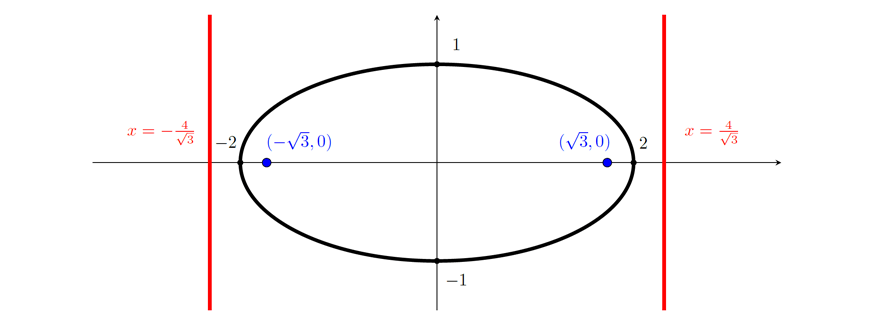### Question 11c

\begin{align*}
\int \frac{1}{x^2 + 10x + 29} dx & = \int \frac{1}{x^2 + 10x + 25 + 4} dx\\
& = \int \frac{1}{(x+5)^2+ 4} dx\\
& = \frac{1}{2}\tan^{-1}\Big(\frac{x+5}{2}\Big)+C
\end{align*}

### Question 11d

We shall first split the integrand into its partial fractions. That is, we wish to find constants $$A$$ and $$B$$ such that $$\frac{6}{x^2-9} = \frac{A}{x+3} + \frac{B}{x-3}.$$ That is, $$6 \equiv A(x-3) + B(x+3).$$
Substituting $$x=3$$ gives us that $$6B=6$$ so $$B=1$$. And similarly, substituting $$x=-3$$ gives us that $$-6A=6$$ so $$A=-1$$.

Hence
\begin{align*}
\int \frac{6}{x^2-9} dx & = \int \frac{-1}{x+3} + \frac{1}{x-3} dx\\
& = -\ln|x+3| + \ln|x-3| +C\\
& = \ln\Big|\frac{x-3}{x+3}\Big| +C
\end{align*}

### Question 11e

Part (i)

$$|z| = \sqrt{(-1)^2 + (\sqrt{3})^2} = \sqrt{1 + 3} = 2$$ and $$\tan \theta = \frac{\sqrt{3}}{-1}$$ so $$arg(z) = \frac{2\pi}{3}$$. Thus, $$z=2cis(\frac{2\pi}{3})$$.

Part (ii)

By De Moivre’s Theorem, $$z^3 = 2^3cis(\frac{2\pi}{3}\cdot 3) = 8cis(2\pi) = 8$$.

### Question 12a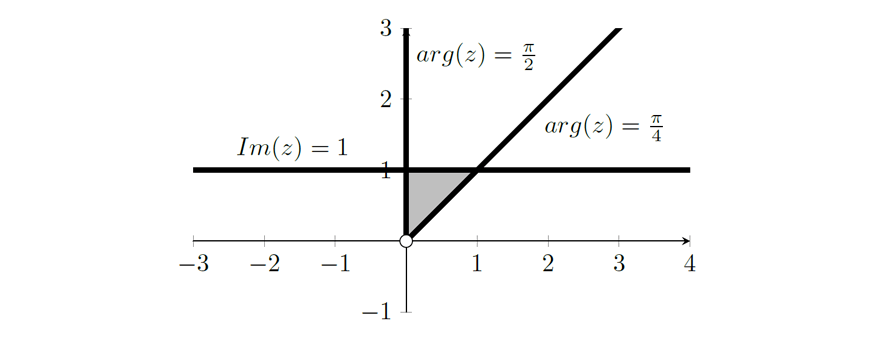### Question 12b

Part (i)

Applying cosine rule on $$\triangle ABP$$, we have that $$70^2 = x^2 + y^2-2\cdot x \cdot y \cdot \cos(\frac{2\pi}{3})$$. Hence, $$70^2 = x^2 +xy+ y^2$$

Part (ii)

As Train $$B$$ is moving at a constant speed of $$4\text{ms}^{-1}$$, $$\frac{dy}{dt}=4$$.

Implicit differentiation on the equation found in part (i) gives us that
\begin{align*}
2x + y + x \frac{dy}{dx} + 2y \frac{dy}{dx}&=0 \\
\frac{dy}{dx}(x+2y)&=-2x-y\\
\frac{dy}{dx}&=\frac{-2x-y}{x+2y}
\end{align*}
Thus, when $$x=30$$ and $$y=50$$, we have that $$\frac{dy}{dx}=\frac{-2\cdot 30 – 50}{30 + 2(50)} = \frac{-110}{130} = \frac{-11}{13}$$.

Finally, we have that $$\frac{dx}{dt} = \frac{dx}{dy}\cdot \frac{dy}{dt} = \frac{13}{-11}\cdot 4 = \frac{-52}{11}$$

### Question 12c

Using the method of cylindrical shells, we have that the radius of the shell would be $$x$$ and that the height of the shell would be $$Ae^{-kx}$$.

Hence, the area of the cylindrical shell at distance $$x$$ is given by:
\begin{align*}
A & = 2\pi r h\\
&= 2 \pi x Ae^{-kx}
\end{align*}
Thus the required volume is given by
\begin{align*}
V &= \int_{10}^{40} 2 \pi x Ae^{-kx} dx\\
&= 2\pi A \int_{10}^{40} x e^{-kx} dx\\
&= 2\pi A \Big[ [x\cdot \frac{1}{-k} e^{-kx}]_{10}^{40} -\int_{10}^{40} \frac{1}{-k} e^{-kx} dx\Big] \text{ (using integration by parts)}\\
&= 2\pi A \Big[ \big(-\frac{40e^{-40k}}{k}+\frac{10e^{-10k}}{k}\big) +\frac{1}{k} \int_{10}^{40} e^{-kx} dx\Big] \\
&= \frac{2\pi A}{k} \Big[ 10e^{-10k}-40e^{-40k} +\frac{1}{-k}[e^{-kx}]_{10}^{40} \Big] \\
&= \frac{2\pi A}{k^2} \Big[ 10ke^{-10k}-40ke^{-40k} -e^{-40k}+e^{-10k} \Big]
\end{align*}

### Question 12d

Part (i)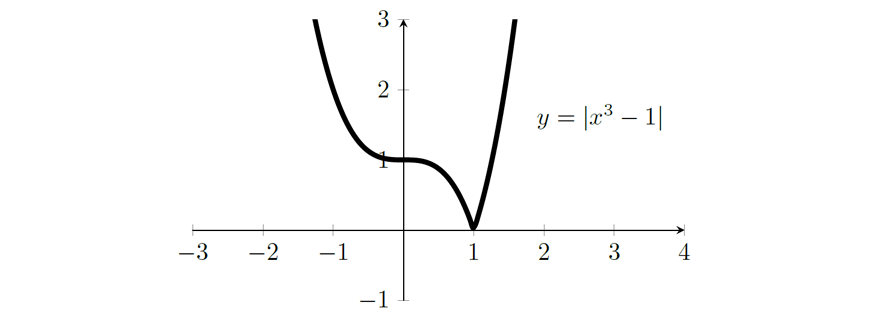Part (ii)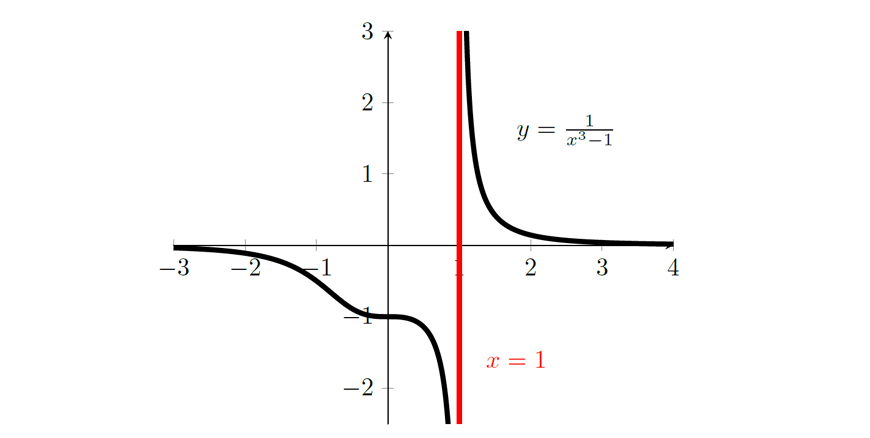Part (iii)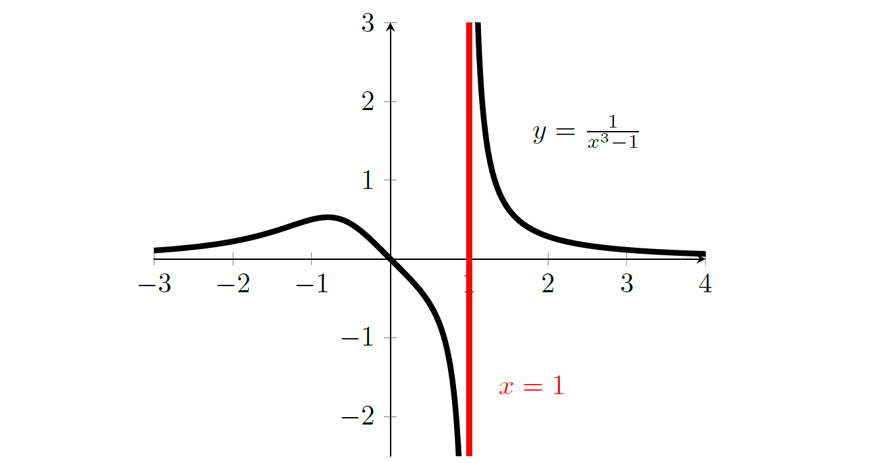### Question 13a

Part (i)

At $$P(a\sec\theta,b\tan\theta)$$, we have that $$\frac{dx}{d\theta}=a\sec\theta\tan\theta$$ and $$\frac{dy}{d\theta}=b\sec^2\theta$$. Hence,
\begin{align*}
\frac{dy}{dx}&=\frac{dy}{d\theta}\cdot \frac{d\theta}{dx}\\
&=\frac{b\sec^2\theta}{a\sec\theta\tan\theta}\\
&=\frac{b\sec\theta}{a\tan\theta}
\end{align*}
Now using the point gradient formula, we have that the equation of the tangent is:
\begin{align*}
y-y_1 &= m(x-x_1)\\
y – b\tan\theta &= \frac{b\sec\theta}{a\tan\theta} (x-a\sec\theta)\\
ay\tan\theta – ab\tan^2\theta &= bx\sec\theta -ab\sec^2\theta\\
ab\sec^2\theta- ab\tan^2\theta &= bx\sec\theta-ay\tan\theta \\
ab(\sec^2\theta- \tan^2\theta) &= bx\sec\theta-ay\tan\theta \\
bx\sec\theta-ay\tan\theta &= ab
\end{align*}

Part (ii)

Tangent at $$P$$:
\begin{equation}
bx\sec\theta-ay\tan\theta = ab \label{eq:1}
\end{equation}
Tangent at $$Q$$:
\begin{equation}
bx\sec\phi-ay\tan\phi = ab \label{eq:2}
\end{equation}
Multiply both sides of $$(1)$$ by $$\tan\phi$$:
\begin{equation}
bx\sec\theta\tan\phi-ay\tan\theta\tan\phi = ab\tan\phi \label{eq:3}
\end{equation}
Multiply both sides of $$(2)$$ by $$\tan\theta$$:
\begin{equation}
bx\sec\phi\tan\theta-ay\tan\phi\tan\theta = ab\tan\theta \label{eq:4}
\end{equation}
The point is intersection is found by solving these equations simultaneously. Thus, by subtracting $$(3)$$ from $$(4)$$, we get that
\begin{align*}
bx_0\sec\phi\tan\theta- bx_0\sec\theta\tan\phi &= ab\tan\theta – ab\tan\phi \\
bx_0(\sec\phi\tan\theta- \sec\theta\tan\phi)&=ab(\tan\theta – \tan\phi)\\
x_0&=\frac{a(\tan\theta – \tan\phi)}{\sec\phi\tan\theta – \sec\theta\tan\phi}
\end{align*}

Part (iii)

To prove that $$O$$, $$M$$ and $$T$$ are collinear, we will show that $$m_{OM} = m_{OT}$$.

First, we find the coordinates of $$M$$.
\begin{align*}
M\Big(\frac{a\sec\theta + a\sec\phi}{2},\frac{b\tan\theta + b\tan\phi}{2}\Big)
\end{align*}
Now we find the gradient $$OM$$.
\begin{align*}
m_{OM} & = \frac{\frac{b\tan\theta + b\tan\phi}{2}-0}{\frac{a\sec\theta + a\sec\phi}{2}-0}\\
& = \frac{b\tan\theta + b\tan\phi}{a\sec\theta + a\sec\phi}\\
& = \frac{b(\tan\theta + \tan\phi)}{a(\sec\theta + \sec\phi)}
\end{align*}

Similarly, we can find the gradient of $$OT$$:
\begin{align*}
m_{OT} & = \frac{\frac{b(\sec\theta – \sec\phi)}{\sec\phi\tan\theta – \sec\theta\tan\phi}-0}{\frac{a(\tan\theta – \tan\phi)}{\sec\phi\tan\theta – \sec\theta\tan\phi}-0}\\
& = \frac{b(\sec\theta – \sec\phi)}{a(\tan\theta – \tan\phi)}
\end{align*}

Now to show that $$m_{OM}=m_{OT}$$, observe that
\begin{align*}
\sec^2\theta – \tan^2\theta &= \sec^2\phi – \tan^2\phi\\
\sec^2\theta – \sec^2\phi &= \tan^2\theta – \tan^2\phi\\
(\sec\theta + \sec\phi)(\sec\theta – \sec\phi) &= (\tan\theta + \tan\phi)(\tan\theta – \tan\phi)\\
\frac{\sec\theta – \sec\phi}{\tan\theta – \tan\phi} &= \frac{\tan\theta + \tan\phi}{\sec\theta + \sec\phi}
\end{align*}
This shows that $$M_{OM} = M_{OT}$$

### Question 13b

Part (i)

As we are finding the area of a trapezium, we need to find the length of the top, bottom and height.

The height is given by the function $$y=1-x^2$$. The using the right-angled isoceles triangle, we see that the length of the base of the trapezium is constant and $$1$$ unit long.

Notice that $$\angle CDA=45^\circ$$. Let $$E$$ be the projection of $$C$$ onto $$AD$$.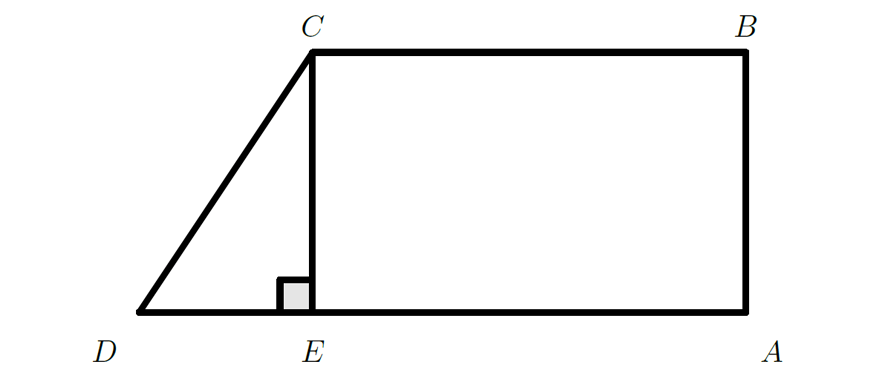Then we have that $$CE=BA=1-x^2$$ and so $$DE=CE=1-x^2$$. This means that $$BC = AD – DE = 1 – (1-x^2) = x^2$$.

Hence, $$Area_{ABCD} = \frac{1+x^2}{2}\cdot (1-x^2) = \frac{1-x^4}{2}$$

Part (ii)

To calculate the volume of the solid, we integrate the area found in (i) from $$-1$$ to $$1$$.
\begin{align*}
V &= \int_{-1}^1 \frac{1-x^4}{2} dx\\
&= 2\int_0^1 \frac{1}{2} – \frac{x^4}{2} dx\\
&= 2\Big[\frac{x}{2} – \frac{x^5}{10}\Big]_0^1\\
&= 2\Big[\frac{1}{2} – \frac{1}{10}-0+0\Big]\\
&= \frac{4}{5} u^3
\end{align*}

### Question 13c

Part (i)

The equations for motion of Object 1 are
\begin{align*}
x &= 20\cos\Big(\frac{\pi}{3}\Big)t\\
x &= 10t\\
\\
y &= -4.9t^2 + 20\sin\Big(\frac{\pi}{3}\Big)t\\
y &= -4.9t^2 + 10\sqrt{3}t
\end{align*}
Hence, Object 1 hits the ground at $$y=0$$, that is, $$-4.9t^2 + 10\sqrt{3}t=0$$ or $$t(-4.9t + 10\sqrt{3})=0$$. This means that $$t = 0$$ or $$t = \frac{10\sqrt{3}}{4.9}$$. But $$t=0$$ is the start of motion, so Object 1 hits the ground at $$t = \frac{10\sqrt{3}}{4.9}$$.

This means that the range is $$x = 10 \cdot \frac{10\sqrt{3}}{4.9} = \frac{100\sqrt{3}}{4.9}$$.

Part (ii)

We are now trying to find the values of $$v$$ and $$\theta$$ that will satisfy the equations of motion for Object 2. Since it is projected two seconds after Object 1, its equations will be, $$x = v\cos\theta(t-2)$$ and $$y = -4.9(t-2)^2 + v\sin\theta(t-2)$$ at the point where $$x=\frac{100\sqrt{3}}{4.9}$$, $$y=0$$ and $$t=\frac{10\sqrt{3}}{4.9}$$. Hence, we have that:
\begin{align}
\frac{100\sqrt{3}}{4.9} &= v\cos\theta\Big(\frac{10\sqrt{3}}{4.9}-2\Big)\nonumber\\
v\cos\theta &= 23.031\ldots \label{eq:5}\\
\nonumber\\
0 &= -4.9\Big(\frac{10\sqrt{3}}{4.9}-2\Big)^2 + v\sin\theta\Big(\frac{10\sqrt{3}}{4.9}-2\Big)\nonumber\\
v\sin\theta &= 7.52050\ldots \label{eq:6}
\end{align}
To solve these simultaneously, divide $$v \sin \theta$$ by $$v \cos \theta$$
\begin{align*}
\tan\theta &= 0.3265381\\
\theta &= 18.1^\circ (1 d.p.)
\end{align*}
Substitute this back to find $$v$$:
\begin{align*}
v\cos18.1^\circ &= 23.031\\
v &= 24.2m/s~(1 d.p.)
\end{align*}

### Question 14a

Let the normal force be $$N$$. Since there are no other forces, resolving the forces gives us:
$$\text{Vertically: } N\cos\theta = mg$$
$$\text{Horizontally: } N\sin\theta = \frac{mv^2}{r}$$

Dividing these equations, we obtain:

\begin{align*}
\frac{N\sin\theta}{N\cos\theta} &= \frac{\frac{mv^2}{r}}{mg}\\
\tan\theta &= \frac{v^2}{rg}\\
v^2 &= gr\tan\theta
\end{align*}

### Question 14b

Part (i)

The terminal velocity is found by solving $$\ddot{x}=0$$. That is,
\begin{align*}
0 &= g-kw\\
w = \frac{g}{k}
\end{align*}

Part (ii)

We require the parachutist to slow down from $$1.6w$$ to $$1.1w$$.
\begin{align*}
\ddot{x} &= g-kv\\
\frac{dv}{dt} &= g-kv\\
\frac{dt}{dv} &= \frac{1}{g-kv}\\
t &= \int_{1.6w}^{1.1w} \frac{1}{g-kv} dv\\
t &= -\frac{1}{k}[ln|g-kv|]_{1.6w}^{1.1w}\\
t &= -\frac{1}{k}\Big[ln|g-1.1kw|-ln|g-1.6kw|\Big]\\
t &= -\frac{1}{k}ln\Big|\frac{g-1.1kw}{g-1.6kw}\Big|\\
t &= -\frac{1}{k}ln\Big|\frac{g-1.1k\frac{g}{k}}{g-1.6k\frac{g}{k}}\Big|\\
t &= -\frac{1}{k}ln\Big|\frac{g-1.1g}{g-1.6g}\Big|\\
t &= -\frac{1}{k}ln\Big|\frac{-0.1g}{-0.6g}\Big|\\
t &= -\frac{1}{k}ln\Big|\frac{1}{6}\Big|\\
t &= \frac{1}{k}ln|6|
\end{align*}

Part (iii)

\begin{align*}
\ddot{x} &= g-kv\\
v\frac{dv}{dx} &= g-kv\\
\frac{dv}{dx} &= \frac{g-kv}{v}\\
\frac{dx}{dv} &= \frac{v}{g-kv}\\
D &= \int_{1.6w}^{1.1w} \frac{v}{g-kv} dv\\
D &= -\frac{1}{k}\int_{1.6w}^{1.1w} \frac{-kv}{g-kv} dv\\
D &= -\frac{1}{k}\int_{1.6w}^{1.1w} 1 -\frac{g}{g-kv} dv\\
D &= -\frac{1}{k}\Big[v + \frac{g}{k}ln|g-kv| \Big]_{1.6w}^{1.1w}\\
D &= \frac{g}{k^2}\Big[\frac{vk}{g} + ln|g-kv| \Big]_{1.1w}^{1.6w}\\
D &= \frac{g}{k^2}\Big[\frac{1.6wk}{g} + ln|g-1.6wk| – \frac{1.1wk}{g} – ln|g-1.1wk| \Big]\\
D &= \frac{g}{k^2}\Big[0.5\frac{vk}{g} + ln\Big|g-1.6k\frac{g}{k}\Big| – ln\Big|g-1.1k\frac{g}{k}\Big| \Big]\\
D &= \frac{g}{k^2}\Big[0.5 + ln\Big|g-1.6g\Big| – ln\Big|g-1.1g\Big| \Big]\\
D &= \frac{g}{k^2}\Big[\frac{1}{2} + ln\Big|-0.6g\Big| – ln\Big|-0.1g\Big| \Big]\\
D &= \frac{g}{k^2}\Big[\frac{1}{2} + ln\Big|\frac{-0.6g}{-0.1g}\Big|\Big]\\
D &= \frac{g}{k^2}\Big[\frac{1}{2} + ln|6|\Big]
\end{align*}

### Question 14c

Part (i)

\begin{align*}
LHS & = \cot x – \cot 2x\\
& = \frac{\cos x}{\sin x} – \frac{\cos 2x}{\sin 2x}\\
& = \frac{\cos x}{\sin x} – \frac{2\cos^2x-1}{2\sin x\cos x}\\
& = \frac{2\cos^2 x}{2\sin x\cos x} – \frac{2\cos^2x-1}{2\sin x\cos x}\\
& = \frac{1}{2\sin x\cos x}\\
& = \frac{1}{\sin 2x}\\
& = \csc 2x
\end{align*}

Part (ii)

Base case: Prove true for $$n=1$$.

$$LHS = \csc 2x$$, $$RHS = \cot x – \cot 2x$$. By part (ii), we see that the statement is true for $$n=1$$.

Inductive step: Assume true for $$n=k$$ where $$k$$ is some integer greater than or equal to 1. That is, assume that $$\sum_{r=1}^k \csc (2^r x) = \cot x – \cot(2^kx).$$
Required to prove true for $$n=k+1$$. That is, we are required to prove that $$\sum_{r=1}^{k+1} \csc (2^r x) = \cot x – \cot(2^{k+1}x).$$
Proof:
\begin{align*}
LHS &= \sum_{r=1}^{k+1} \csc (2^r x)\\
&= \sum_{r=1}^{k} [\csc (2^r x)] + \csc (2^{k+1} x)\\
&= \cot x – \cot(2^kx) + \csc (2^{k+1} x)\\
&= \cot x – \cot (2^{k+1} x) \text{ by substituting } x \text{ for } 2^kx \text{ in part (i)}\\
&= RHS
\end{align*}

### Question 15a

Part (i)

\begin{align*}
RHS &= \int_{-a}^a\frac{f(-x)}{f(x) + f(-x)} dx\\
&= \int_{a}^{-a}\frac{f(u)}{f(-u) + f(u)} \cdot -du \text{ substituting } u = -x\\
&= \int_{-a}^{a}\frac{f(u)}{f(-u) + f(u)} \cdot du\\
&= \int_{-a}^{a}\frac{f(x)}{f(-x) + f(x)} \cdot dx \text{ replacing } u \text{ with } x\\
&= LHS
\end{align*}

Part (ii)

Let $$I = \int_{-1}^1\frac{e^{x}}{e^x + e^{-x}} dx.$$ Then by part (i) we also have that $$I = \int_{-1}^1\frac{e^{-x}}{e^x + e^{-x}} dx.$$ Adding the two equations above, we have that
\begin{align*}
2I &= \int_{-1}^1\frac{e^{x}}{e^x + e^{-x}} +\int_{-1}^1\frac{e^{-x}}{e^x + e^{-x}} dx \\
2I &= \int_{-1}^1\frac{e^{x} + e^{-x}}{e^x + e^{-x}} dx\\
2I &= \int_{-1}^1 1 dx \\
2I &= 2 \\
I &= 1
\end{align*}

### Question 15b

Part (i)

In the case where $$w=y$$, the chance for either play to win on their turn is the same. Since, player $$A$$ goes first, they will have a higher chance of winning.

Part (ii)

Note that as the game progresses, the number of balls in the urn never changes until there is a winner. Thus, the probability that $$A$$ will win on any turn is $$\frac{w}{w+y}$$ and the probability that $$B$$ will win on any turn is $$\frac{y}{w+y}.$$
The cases where $$B$$ will win the game are:

• $$A$$ loses, $$B$$ wins with probability $$\frac{y}{w+y} \cdot \frac{y}{w+y} = \big(\frac{y}{w+y}\big)^2$$
• $$A$$ loses, $$B$$ loses, $$A$$ loses, $$B$$ wins with probability $$\frac{y}{w+y} \cdot \frac{w}{w+y} \cdot \frac{y}{w+y} \cdot \frac{y}{w+y} = \big(\frac{w}{w+y}\big)\big(\frac{y}{w+y}\big)^3$$
• $$A$$ loses, $$B$$ loses, $$A$$ loses, $$B$$ loses, $$A$$ loses, $$B$$ wins with probability $$\big(\frac{w}{w+y}\big)^2\big(\frac{y}{w+y}\big)^4$$
• And so on…

Summing these together, we find that the probability that $$B$$ will win is
\begin{align*}
P(B~wins) &= \big(\frac{y}{w+y}\big)^2 + \big(\frac{w}{w+y}\big)\big(\frac{y}{w+y}\big)^3 + \big(\frac{w}{w+y}\big)^2\big(\frac{y}{w+y}\big)^4 + \cdots \\
P(B~wins) &= \frac{\big(\frac{y}{w+y}\big)^2}{1-\big(\frac{w}{w+y}\big)\big(\frac{y}{w+y}\big)}\\
P(B~wins) &= \frac{y^2}{(w+y)^2 – wy}\\
P(B~wins) &= \frac{y^2}{w^2 + 2wy + y^2 – wy}\\
P(B~wins) &= \frac{y^2}{w^2 + wy + y^2}
\end{align*}
In order for $$B$$ to have a greater chance of winning, we need $$P(B~win) > \frac{1}{2}$$. That is,
\begin{align*}
\frac{y^2}{w^2 + wy + y^2} > \frac{1}{2}\\
2y^2 > w^2 + wy + y^2\\
y^2 – wy – w^2 > 0\\
\Big(\frac{y}{w}\Big)^2 – \frac{y}{w} – 1 > 0
\end{align*}
Thus, $$\frac{y}{w} > \frac{1 + \sqrt{1+4}}{2} \text{ or } \frac{y}{w} < \frac{1 – \sqrt{1+4}}{2}$$
$$\frac{y}{w} > \frac{1 + \sqrt{5}}{2} \text{ or } \frac{y}{w} < \frac{1 – \sqrt{5}}{2}.$$
But $$w$$ and $$y$$ are positive, so we must have that $$\frac{y}{w} > \frac{1 + \sqrt{5}}{2}$$

### Question 15c

Part (i)

\begin{align*}
\int_0^1 \frac{x}{(x+1)^2} dx &= \int_0^1 \frac{x+1 -1}{(x+1)^2} dx\\
&= \int_0^1 \frac{x+1}{(x+1)^2} – \frac{1}{(x+1)^2} dx\\
&= \int_0^1 \frac{1}{x+1} – \frac{1}{(x+1)^2} dx\\
&= \Big[\ln|x+1| + \frac{1}{x+1}\Big]_{0}^1\\
&= \Big[\ln|2| + \frac{1}{2}\Big] – \Big[\ln|1| + \frac{1}{1}\Big]\\
& = \ln2 – \frac{1}{2}
\end{align*}

Part (ii)

\begin{align*}
I_n &= \int_0^1\frac{x^n}{(x+1)^2}dx\\
&=\int_0^1\frac{x^{n-1}((x+1)-1)}{(x+1)^2}dx\\
&=\int_0^1\frac{x^{n-1}}{x+1}dx – I_{n-1}
\end{align*}

 $$u = (x+1)^{-1}$$ $$v’ = x^{n-1}$$ $$u’ = -\frac{1}{(x+1)^2}$$ $$v = \frac{x^n}{n}$$

\begin{align*}
∴ I_n &= \left[\frac{x^n}{x(1+x)}\right]_0^1 + \frac{1}{n}\int_0^1\frac{x^n}{(1+x)^2}dx – I_{n-1}\\
&= \frac{1}{2n} + \frac{1}{n}I_n – I_{n-1}\\
∴ I_n&(1-\frac{1}{n}) = \frac{1}{2n}-I_{n-1}\\
I_n&(\frac{n-1}{n}) = \frac{1}{2n} – I_{n-1}\\
I_n &= \frac{1}{2(n-1)} – \frac{n}{n-1}I_{n-1} \,\, \textrm{ as required}
\end{align*}

Part (iii)

\begin{align*}
I_3 &= \frac{1}{2(2)} – \frac{3}{2}I_2\\
&= \frac{1}{4} – \frac{3}{2}(\frac{1}{2}-2I_1)\\
&= \frac{1}{4} – \frac{3}{4} + 3(\ln 2 – \frac{1}{2})\\
&= -\frac{1}{2} + 3\ln 2 – \frac{3}{2}\\
&= 3\ln 2 – 2
\end{align*}

### Question 16a

Part (i)

\begin{align*}
x^3+px+q=0, \,\,p,q\in\mathbb{R}\,\,p>0\\
\textrm{Letting }r=\sqrt{\frac{4p}{3}} \textrm{ and } \cos 3\theta = -\frac{4q}{r^3}:\\
\textrm{Now }-\frac{4q}{r^3} = 4\cos^3\theta – 3\cos\theta\\
∴ r^3\cos^3\theta – \frac{3r^2}{4}(r\cos\theta)+q = 0\\
\textrm{Since } r=\sqrt{\frac{4p}{3}},\,\,p = \frac{3r^2}{4}\\
\end{align*}

Therefore the above is a polynomial in $$r\cos\theta$$ in the form $$x^3+px+q = 0$$ and hence $$r\cos\theta$$ is a root.

Part (ii)

\begin{align*}
x^3+9x^2+15x-17&=0\\
\textrm{Let }u=\alpha+3, \textrm{ so }\alpha = u&-3\\
\textrm{Then } (u-3)^3 + 9(u-3)^2 + 15(u-3) – 17 &= 0\\
u^3-9u^2+27u-27 + 9u^2 – 54u + 81 + 15u – 45 – 17 &= 0\\
u^3 – 12u – 8 &= 0\\
\textrm{Hence } \alpha+3,\beta+3,\gamma+3 \textrm{ are roots of } x^3-12x-8&=0
\end{align*}

Part (iii)

Solve $$x^3-12x-8=0$$ for $$\alpha+3,\beta+3,\gamma+3$$ by recognising that it is of the form in part (i) where $$p=12$$ and $$q=-8$$.
ie. $$r=\sqrt{\frac{4\times12}{3}} = 4$$ and $$\cos3\theta = -\frac{4(-8)}{4^3}=\frac{1}{2}$$
The roots will be of the form $$4\cos\theta$$ where $$\cos3\theta = \frac{1}{2}$$
$$\Rightarrow3\theta = \frac{\pi}{3},\frac{5\pi}{3},\frac{7\pi}{3}\Rightarrow \theta = \frac{\pi}{9},\frac{5\pi}{9},\frac{7\pi}{9}$$
$$∴ \alpha+3 = 4\cos\frac{\pi}{9}, \beta+3 = \frac{5\pi}{9}, \gamma+3 = \frac{7\pi}{9}$$
and thus the solutions of $$x^3+9x^2+15x-17=0$$ are $$4\cos\frac{\pi}{9} – 3, 4\cos\frac{5\pi}{9}-3, 4\cos\frac{7\pi}{9}-3$$

### Question 16b

Part (i)

\begin{align*}
\textrm{Let } P(z) = z^4 – 2kz^3 + 2k^2z^2 + mz + 1\\
\textrm{where } k,m\in\mathbb{R}.\textrm{ Roots are } \alpha,\bar{\alpha},\beta,\bar{\beta}.\,\,|\alpha|=|\beta|=1\\
\end{align*}

We use the fact that:

\begin{align*}
(Re(\alpha))^2 + (Re(\beta))^2 &= (Re(\alpha) + Re(\beta))^2 – 2Re(\alpha)Re(\beta)\\
\textrm{where } Re(\alpha)+ Re(\beta) &= \frac{\alpha+\bar{\alpha}+\beta+\bar{\beta}}{2} = \frac{2k}{2} = k\\
\textrm{and } 2Re(\alpha)Re(\beta) &= 2\left(\frac{\alpha+\bar{\alpha}}{2}\right)\left(\frac{\beta+\bar{\beta}}{2}\right)\\
&=\frac{1}{2}(\alpha\beta + \alpha\bar{\beta} + \bar{\alpha}\beta + \bar{\alpha}\bar{\beta})\\
&=\frac{1}{2}[(\alpha\beta + \alpha\bar{\beta} + \bar{\alpha}\beta + \bar{\alpha}\bar{\beta} + \alpha\bar{\alpha} + \beta\bar{\beta}) – (\alpha\bar{\alpha} + \beta\bar{\beta})]\\
&=\frac{1}{2}(2k^2-2)\\
&=k^2-1\\
\textrm{Thus } (Re(\alpha))^2 + (Re(\beta))^2 &= k^2-(k^2-1) = 1 \textrm{ as required}\\
\end{align*}

Part (ii)

From part (i) we have that $$Re(\alpha)^2 + Re(\beta)^2 = 1$$. But since $$|a|=1$$ we have that $$Re(\alpha)^2 + Im(\alpha)^2 = 1$$. Hence, we must have $$Re(\beta)^2 = Im(\alpha)^2$$ so that $$Re(\beta)^2 = \pm Im(\alpha)$$. Since $$|\beta|=1$$ we have that $$\beta$$ also lies on the unit circle. Hence, plotting the possible positions of $$\beta$$, we have that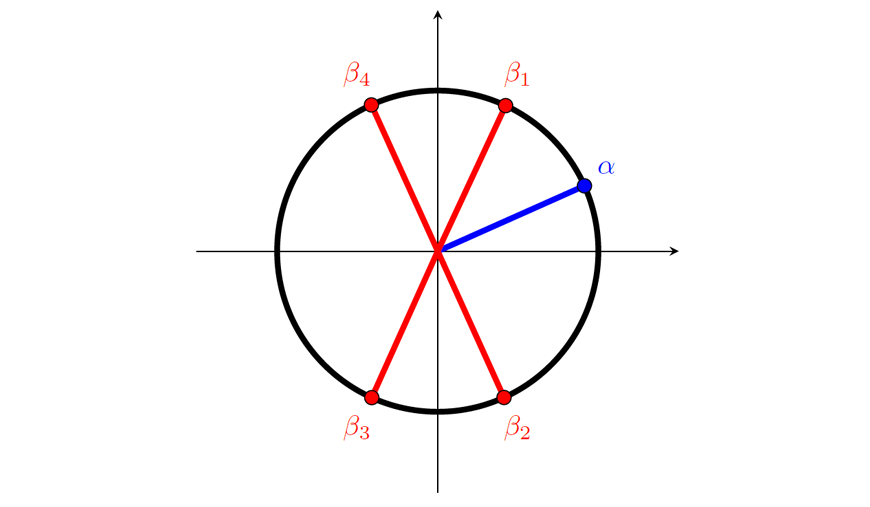### Question 16c

\begin{align*}
\angle ACD &= \angle ABD \textrm{ (angles standing on common arcs)}\\
\angle BAC &= \angle BDC \textrm{ (angles standing on common arcs)}\\
\angle ACD &= \pi – \angle AED \textrm{ (opposite angles on cyclic quad)}\\
∴ \angle ABD &= \pi – E\\
∴ B &= \angle ABD + \angle CBD = \angle CBD + \pi – E\\
∴ \angle CBD &= B + E – \pi\\
C &= \angle DCA + \angle ACB\\
&= \pi – E + \angle ACB \textrm{ (opposite angles on cyclic quad)}\\
∴ \angle ACB &= C + E – \pi\\
\textrm{Consider } \Delta ABC &\textrm{ and } \Delta BCD\\
\frac{a}{\sin\angle CBD}&=\frac{e}{\sin\angle BDC}\\
∴ e &= \frac{a\sin\angle BDC}{\sin\angle CBD}\\
\frac{d}{\sin\angle ACB}&=\frac{e}{\sin\angle BAC}\\
∴ e &= \frac{d\sin\angle BAC}{\sin\angle ACB}\\
∴ \frac{a\sin\angle BDC}{\sin\angle CBD} &= \frac{d\sin\angle BAC}{\sin\angle ACB}\\
\textrm{Since } \angle BAC &= \angle BDC\\
\sin\angle BAC &= \sin\angle BDC\\
∴ \frac{a}{\sin\angle CBD} &= \frac{d}{\sin\angle ACB}\\
\frac{a}{\sin(B+E-\pi)} &= \frac{d}{\sin(C+E-\pi)}\\
\frac{a}{-\sin(\pi-(B+E))} &= \frac{d}{-\sin(\pi-(C+E))}\\
\frac{a}{\sin(B+E)} &= \frac{d}{\sin(C+E)} \textrm{ as required}
\end{align*}

## Want to know your ATAR?

Use the calculator to explore the HSC Marks you need to achieve your ATAR Goal.